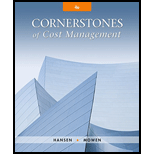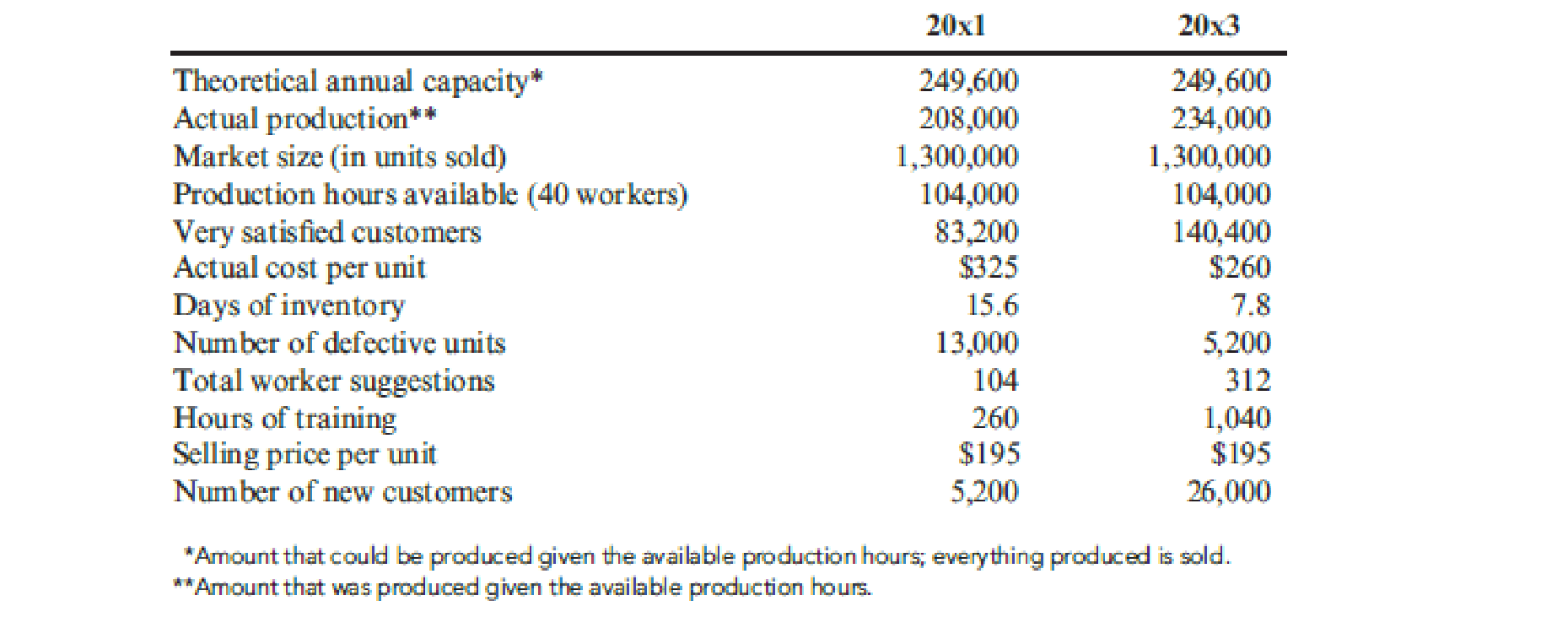# At the end of 20x1, Mejorar Company implemented a low-cost strategy to improve its competitive position. Its objective was to become the low-cost producer in its industry. A Balanced Scorecard was developed to guide the company toward this objective. To lower costs, Mejorar undertook a number of improvement activities such as JIT production, total quality management, and activity-based management. Now, after two years of operation, the president of Mejorar wants some assessment of the achievements. To help provide this assessment, the following information on one product has been gathered: Required: 1. Compute the following measures for 20x1 and 20x3: a. Actual velocity and cycle time b. Percentage of total revenue from new customers (assume one unit per customer) c. Percentage of very satisfied customers (assume each customer purchases one unit) d. Market share e. Percentage change in actual product cost (for 20x3 only) f. Percentage change in days of inventory (for 20x3 only) g. Defective units as a percentage of total units produced h. Total hours of training i. Suggestions per production worker j. Total revenue k. Number of new customers 2. For the measures listed in Requirement 1, list likely strategic objectives, classified according to the four Balance Scorecard perspectives. Assume there is one measure per objective.### Cornerstones of Cost Management (C...

4th Edition
Don R. Hansen + 1 other
Publisher: Cengage Learning
ISBN: 9781305970663

#### Solutions

Chapter
Section### Cornerstones of Cost Management (C...

4th Edition
Don R. Hansen + 1 other
Publisher: Cengage Learning
ISBN: 9781305970663
Chapter 13, Problem 21P
Textbook Problem
346 views

## At the end of 20x1, Mejorar Company implemented a low-cost strategy to improve its competitive position. Its objective was to become the low-cost producer in its industry. A Balanced Scorecard was developed to guide the company toward this objective. To lower costs, Mejorar undertook a number of improvement activities such as JIT production, total quality management, and activity-based management. Now, after two years of operation, the president of Mejorar wants some assessment of the achievements. To help provide this assessment, the following information on one product has been gathered:Required: 1. Compute the following measures for 20x1 and 20x3: a. Actual velocity and cycle time b. Percentage of total revenue from new customers (assume one unit per customer) c. Percentage of very satisfied customers (assume each customer purchases one unit) d. Market share e. Percentage change in actual product cost (for 20x3 only) f. Percentage change in days of inventory (for 20x3 only) g. Defective units as a percentage of total units produced h. Total hours of training i. Suggestions per production worker j. Total revenue k. Number of new customers 2. For the measures listed in Requirement 1, list likely strategic objectives, classified according to the four Balance Scorecard perspectives. Assume there is one measure per objective.

1. a

To determine

Compute the actual velocity and cycle time for 20x1 and 20x3.

### Explanation of Solution

Cycle time and velocity: “Cycle time and velocity are two operational “measures of responsiveness”. Cycle time is the spans of time taken to produce a unit of output from the time of receipt of materials till the good is supplied to finished goods inventory. Therefore, cycle time is the time taken to produce a product”. Velocity is the number of units of output that could be produced within a given period of time”

Strategy Translation: Strategy translation is the process of specifying objectives, measures, targets, and initiatives for each individual perspective. Strategic focus is created by translating the organizations’ strategy into operational objectives and performance measures for four different perspectives.

Calculate actual velocity for 20x1:

Actualvelocity=ActualproductionProductionhoursavailablefor40workers=208,000units104,000hours=2unitsperhour

Calculate cycle time for 20x1:

Cycletime=TimetakenUnitsproduced=60minutes2

b.

To determine

Calculate percentage of total revenue from new customers:

c.

To determine

Calculate the percentage of satisfied customers.

d.

To determine

Calculate value of market share.

e.

To determine

Calculate percentage change in actual product cost for 20x3.

f.

To determine

Calculate percentage change in days of inventory for 20x3.

g.

To determine

Calculate the defective units as a percentage of total units produced.

h.

To determine

Calculate the total hours of training.

i.

To determine

Calculate per production worker.

j.

To determine

Calculate total revenue.

k.

To determine

Identify the number of new customers during 20x1 and 20x3.

2.

To determine

Provide strategic objective classified according to the four balance scorecard perspectives.

### Still sussing out bartleby?

Check out a sample textbook solution.

See a sample solution

#### The Solution to Your Study Problems

Bartleby provides explanations to thousands of textbook problems written by our experts, many with advanced degrees!

Get Started